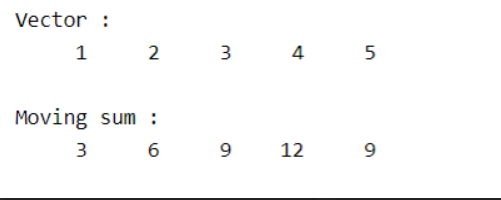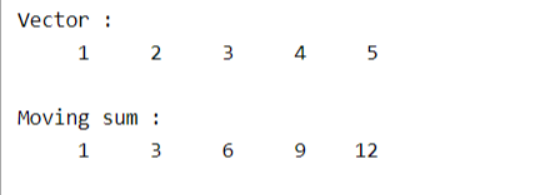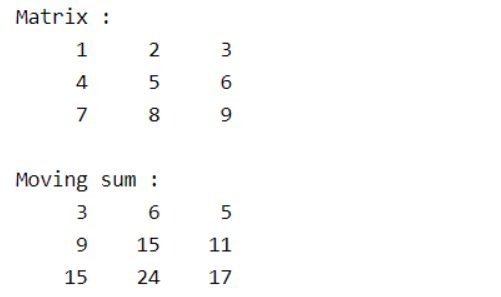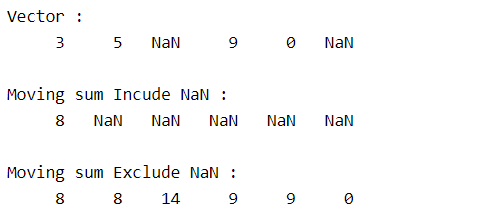Related Articles

# How to Calculate Moving Sum in MATLAB?

• Last Updated : 14 May, 2021

The moving sum of a vector can be referred to as the running sum of each window of some size k in the vector.

Suppose vector = [1 2 3 4 5]

Then the moving sum of each window of size 2 are

[1 2] = 3

[2 3] = 5

[3 4] = 7

[4 5] = 9

Then vector of moving sums is [3 5 7 9]

Matlab allows us to perform this operation effectively using movsum() method. Different syntaxes associated with movsum() method are:

• M = movsum(A, k)
• M = movsum(A, [kb kf])
• M = movsum(___, dim)
• M = movsum(___, nanflag)

Let us now discuss the above syntax in detail:

### movsum(A, k)

• The method returns an array of k-size window sums of vector A.
• The returned vector will have the same size as A.
• If k is odd, then the window is centered about the element in the current position.

Suppose A = [1 2 3 4 5] and k = 3, then sliding window size is 3.

Since k is odd, window is centered at every index and considers one value from left and one value from right into a window.

Then windows are

[1 2] –> Since at first index(i.e value 1) there is no left value, then window gets truncated to size 2

[1 2 3]

[2 3 4]

[3 4 5]

[4 5] —> Since at last index(i.e value 5) there is no right value, then window gets truncated to size 2

• If k is even, the window is centered on the current and previous elements.
• When there are few elements in the window, then the window automatically gets truncated and considers the sum of the truncated window.

## Matlab

 `% Input vector``A = [1 2 3 4 5];``disp(``"Vector :"``);``disp(A);`` ` `% Moving sum of A of size 3``M = movsum(A,3);``disp(``"Moving sum :"``);``disp(M);`

Output :### movsum(A, [kb kf])

Returns the moving sum of vector A, with every window, is centered at the present position and having left side kb elements and right side kf elements having a total window of size kb+kf+1.

Suppose A = [1 2 3 4 5] and [kb = 2 kf = 0], then sliding window size is kb+kf+1 = 3

At every element previous 2 elements and next 0 elements are considered into the window along with current element.

Then windows are



[1 2]

[1 2 3]

[2 3 4]

[3 4 5]

## Matlab

 `% Input vector``A = [1 2 3 4 5];``disp(``"Vector :"``);``disp(A);`` ` `% Moving sum of A of size 3``% every window having 2 elements ``% to left  and 0 elements to the ``% right``M = movsum(A,[2 0]);``disp(``"Moving sum :"``);``disp(M);`

Output :### movsum(___,dim)

• Returns the moving sum of matrix by calculating across each dimension of dim.
• dim takes two values 1 or 2 corresponds to operate along each column and along each row respectively.
• The default value of dim = 1, i.e perform moving sum of matrix across each column.

## Matlab

 `% Input vector``A = [1 2 3; 4 5 6; 7 8 9];``disp(``"Matrix :"``);``disp(A);`` ` `% Moving sum of A of size k=3 ``% along each row(dim=1)``M = movsum(A,3,2);``disp(``"Moving sum :"``);``disp(M);`

Output :### movsum(___,nanflag)

• nanflag value decides whether to include or exclude NaN value of the vector in moving sum or not.
• nanflag takes two values ‘includenan’ or ‘omitnan’ corresponds to including NaN elements and excluding NaN elements respectively.
• omitNaN’ considers NaN values as 0.

Note : NaN + number = NaN

## Matlab

 `% Input vector``A = [3 5 NaN 9 0 NaN];``disp(``"Vector :"``);``disp(A);`` ` `% Including NaN values ``B = movsum(A,3,``'includenan'``);``disp(``"Moving sum Incude NaN :"``);``disp(B);`` ` `% Excluding NaN values ``B = movsum(A,3,``'omitnan'``);``disp(``"Moving sum Exclude NaN :"``);``disp(B);`

Output :My Personal Notes arrow_drop_up